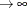The QLIM Procedure

Automated MCMC

Subsections:

The main purpose is to provide the user with the opportunity of obtaining a good approximation of the posterior distribution without initializing the MCMC algorithm: initial values, proposal distributions, burn-in and number of samples.

The automated algorithm is composed of two phases: tuning and sampling. In the tuning phase, there are two main concerns: the choice of a good proposal distribution and the search for the stationary region of the posterior distribution. In the sampling phase, the algorithm will decide how many samples are necessary to obtain good approximations of the posterior mean and some quantiles of interest.

Tuning Phase

During the tuning phase, the algorithm tries to search for a good proposal distribution and, at the same time, to reach the stationary region of the posterior. The choice of the proposal distribution is based on the analysis of the acceptance rates. This is similar to what is done in PROC MCMC: for more details see Tuning the Proposal Distribution: Tuning the Proposal Distribution in SAS/STAT 13.2 User's Guide. For the stationarity analysis, the main idea is to run two tests, Geweke (Ge) and Heidleberger-Welch (HW), on the posterior chains at the end of each attempt. For more details, see Geweke Diagnostics: Geweke Diagnostics in SAS/STAT 13.2 User's Guide, and Heidelberger and Welch Diagnostics: Heidelberger and Welch Diagnostics in SAS/STAT 13.2 User's Guide. If the stationarity hypothesis is rejected, then the tuning samples are increased and the tests repeated in the next attempt. After 10 attempts, the tuning phase will be ended regardless of the results. The tuning parameters for the first attempt are fixed: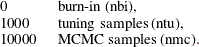For the remaining attempts, the tuning parameters will be adjusted dynamically. More specifcally, each parameter will be assigned an acceptance ratio (AR) of the stationarity hypothesis: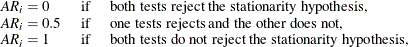for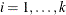. Then, an overall stationarity average (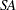) for all parameters ratios is evaluated,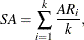and the number of tuning samples is updated accordingly: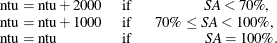The Heidelberger-Welch test also provides an indications of how much burn-in should be used. The algorithm requires this burn-in to be: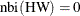. If that is not the case, the burn-in will updated accordingly,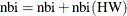, and a new tuning attempt will be implemented. This choice is motivated by the fact that the burn-in must be discarded in order to reach the stationary region of the posterior distribution.

The number of samples predicted by the Raftery-Lewis nmc(RL) diagnostic tool will be also taken into consideration: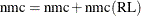. For more details see Raftery and Lewis Diagnostics: Raftery and Lewis Diagnostics in SAS/STAT 13.2 User's Guide. However, in order to exit the tuning phase, it will not be required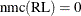.

Sampling Phase

The main idea of the sampling phase is to make sure that the mean and a quantile of interest are evaluated accurately. This can be tested by implementing the half-width test by Heidelberger-Welch and by analyzing the Raftery-Lewis diagnostic tool. In addition, the requirements defined in the tuning phase will also be checked: the Geweke and the Heidelberger-Welch tests must not reject the stationary hypothesis and the burn-in predicted by the Heidelberger-Welch test must be zero.

The sampling phase is characterized by a maximum of 10 attempts. If the algorithm exceeds this limit, the sampling phase will end and indications on how to improve sampling will be given. The sampling phase will first update the burn-in with the information provided by the HW test:. Then, it determines the difference between the actual number of samples and the number of samples predicted by the RL test: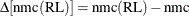. The new number of samples will be updated accordingly: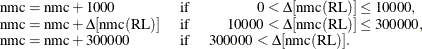Finally, the HW half-width test checks whether the sample mean is accurate. If the mean of any parameters is not considered to be accurate, the number of sampling is increased: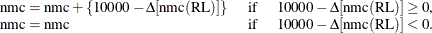Standard Distributions

Table 22.3 through Table 22.8 show all the distribution density functions that PROC QLIM recognizes. You specify these distribution densities in the PRIOR statement.

Table 22.3: Beta Distribution

 PRIOR statement BETA(SHAPE1=a, SHAPE2=b, MIN=m, MAX=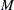) Note: Commonly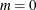and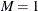. Density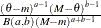Parameter restriction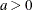,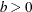,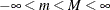Range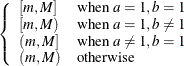Mean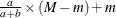Variance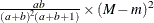Mode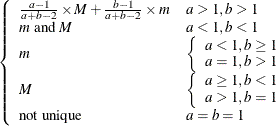Defaults SHAPE1=SHAPE2=1,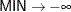,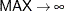Table 22.4: Gamma Distribution

 PRIOR statement GAMMA(SHAPE=a, SCALE=b ) Density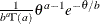Parameter restriction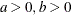Range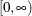Mean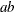Variance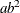Mode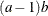Defaults SHAPE=SCALE=1

Table 22.5: Inverse-Gamma Distribution

 PRIOR statement IGAMMA(SHAPE=a, SCALE=b) Density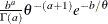Parameter restrictionRange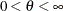Mean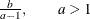Variance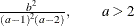Mode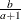Defaults SHAPE=2.000001, SCALE=1

Table 22.6: Normal Distribution

 PRIOR statement NORMAL(MEAN=, VAR=) Density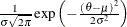Parameter restriction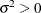Range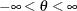MeanVarianceModeDefaults MEAN=0, VAR=1000000

Table 22.7: t Distribution

 PRIOR statement T(LOCATION=, DF=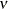) Density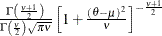Parameter restriction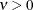RangeMean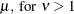Variance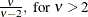ModeDefaults LOCATION=0, DF=3

Table 22.8: Uniform Distribution

 PRIOR statement UNIFORM(MIN=m, MAX=) Density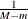Parameter restrictionRange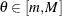Mean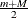Variance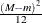Mode Not unique Defaults MIN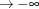, MAX